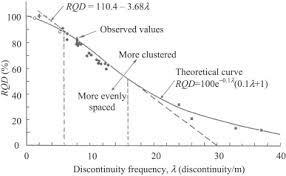## How to Calculate and Solve for Rock Density Designation | Rock MechanicsThe image above represents rock quality designation.

To compute for rock quality designation, two essential parameters are needed and these parameters are Sum of the Length of Core Pieces > 10cm (S) and Total Length Drilled or Core Recovery (L).

The formula for calculating the rock quality designation:

RQD = 100(S / L)

Where:

RQD = Rock Quality Designation
S = Sum of the Length of Core Pieces > 10cm
L = Total Length Drilled or Core Recovery

Let’s solve an example;
Calculate the rock quality designation? Given that the sum of the length of core pieces > 10cm is 20 and the total length drilled or core recovery is 14.

This implies that;

S = Sum of the Length of Core Pieces > 10cm = 20
L = Total Length Drilled or Core Recovery = 14

RQD = 100(S / L)
RQD = 100(20 / 14)
RQD = 100(1.428)
RQD = 142.85

Therefore, the rock quality designation is 142.85.

Calculating the Sum of the Length of Core Pieces > 10cm when the Rock Quality Designation and the Total Length Drilled or Core Recovery is Given.

S = RQD x L

Where:

S = Sum of the Length of Core Pieces > 10cm
RQD = Rock Quality Designation
L = Total Length Drilled or Core Recovery

Let’s solve an example;
Calculate the sum of the length of core pieces > 10cm? Given that the rock quality designation is 32 and the total length drilled or core recovery is 11.

This implies that;

RQD = Rock Quality Designation = 32
L = Total Length Drilled or Core Recovery = 11

S = RQD x L
S = 32 x 11
S = 352

Therefore, the sum of the length of core pieces > 10cm is 352.